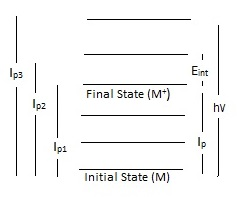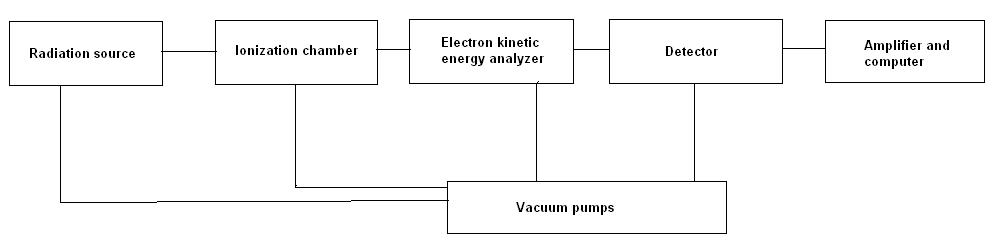# Photoelectron Spectroscopy

$$\newcommand{\vecs}{\overset { \rightharpoonup} {\mathbf{#1}} }$$ $$\newcommand{\vecd}{\overset{-\!-\!\rightharpoonup}{\vphantom{a}\smash {#1}}}$$$$\newcommand{\id}{\mathrm{id}}$$ $$\newcommand{\Span}{\mathrm{span}}$$ $$\newcommand{\kernel}{\mathrm{null}\,}$$ $$\newcommand{\range}{\mathrm{range}\,}$$ $$\newcommand{\RealPart}{\mathrm{Re}}$$ $$\newcommand{\ImaginaryPart}{\mathrm{Im}}$$ $$\newcommand{\Argument}{\mathrm{Arg}}$$ $$\newcommand{\norm}{\| #1 \|}$$ $$\newcommand{\inner}{\langle #1, #2 \rangle}$$ $$\newcommand{\Span}{\mathrm{span}}$$ $$\newcommand{\id}{\mathrm{id}}$$ $$\newcommand{\Span}{\mathrm{span}}$$ $$\newcommand{\kernel}{\mathrm{null}\,}$$ $$\newcommand{\range}{\mathrm{range}\,}$$ $$\newcommand{\RealPart}{\mathrm{Re}}$$ $$\newcommand{\ImaginaryPart}{\mathrm{Im}}$$ $$\newcommand{\Argument}{\mathrm{Arg}}$$ $$\newcommand{\norm}{\| #1 \|}$$ $$\newcommand{\inner}{\langle #1, #2 \rangle}$$ $$\newcommand{\Span}{\mathrm{span}}$$$$\newcommand{\AA}{\unicode[.8,0]{x212B}}$$

Photoelectron spectroscopy involves the measurement of kinetic energy of photoelectrons to determine the binding energy, intensity and angular distributions of these electrons and use the information obtained to examine the electronic structure of molecules. It differs from the conventional methods of spectroscopy in that it detects electrons rather than photons to study electronic structures of a material.

## Introduction

Photoelectron spectroscopy (PES) is the energy measurements of photoelectrons emitted from solids, gases, or liquids by the photoelectric effect. Depending on the source of ionization energy, PES can be divided accordingly into Ultraviolet Photoelectron Spectroscopy (UPS) and X-ray Photoelectron Spectroscopy (XPS). The source of radiation for UPS is a noble gas discharge lamp, usually a He discharge lamp. For XPS, also referred to as Electron Spectroscopy for Chemical Analysis (ESCA), the source is high energy X-rays (1000-1500 eV). Furthermore, depending on the source of the ionization energy, PES can probe either valence or core electrons. UPS, which uses the energy of ultraviolet rays (<41 eV) will only be sufficient to eject electrons from the valence orbitals, while the high energy X-rays used in XPS can eject electrons from the core and atomic orbitals (Figure 1).Figure 1. Scheme for UPS and XPS. Illustrates the ejection of electrons from respectively the valence electrons and core electrons.1

Further information, about XPS and UPS, is discussed within the module on Photoelectron Spectroscopy: Application, which includes a discussion on methods of study for both these spectroscopic techniques and a comparison.

## Photoelectric Effect

To understand the principles of photoelectron spectroscopy, the photoelectric effect must be applied. The photoelectric effect states that electrons can be pushed off the surface of a solid by electromagnetic radiation. The ejected electrons are called photoelectrons.Figure 2. Scheme of photoelectric effect. Incoming light hits the surface of a solid causing the ejection of a photoelectron.

Originally, known as the Hertz effect, the photoelectric effect was first observed by Heinrich Hertz in 1887, when Hertz noticed that sparks would more readily jump between two charged surfaces that were illuminated with light. Hertz’s observation would ultimately lead to Einstein’s photoelectric law; the kinetic energy of an emitted photoelectron is given by

$E_k = h\nu - E_I \label{1}$

where h is Planck’s constant, ν is the frequency of the ionizing light, and EI is the ionization energy, which is synonymous with electron binding energy, of the electron. The term photoelectric effect is only considered when discussing solids, exclusively. As PES can be used for energy measurements of solids, liquids, and gases the term photoionization or photoemission better represents the principles of PES. Photoionization is the process in which molecule (M) is ionized by a beam of photons, in which the molecule will lose an electron:

$M + h\nu \rightarrow M^{^{+}}(E_{int}) + e \label{2}$

This process of photoionization follows the three-step model. The three-step model breaks down the process of photoionization into three independent steps:

1. The molecule will absorb the photon, causing the energy of the photon to be transferred to the molecule's electrons, which will become excited.
2. The excited electron will travel to the surface of the molecule. During this step, the excited electron travels it may or may not collide with other particles. Any excited electrons which do collide with a particle will loss energy.
3. The excited electron will escape the surface of the molecule into the vacuum where it will be detected.

The process of photoionization can only occur if the photon has energy greater than the energy which is holding the electron to the molecule, which is the lowest ionization potential. If there is excess energy after the ionization has occurred then the excess energy will be in the form of kinetic energy. By rearranging equation 1, the ionization energy will be the difference between the photon energy (hv) and the photoelectron's kinetic energy (Ek). These two variables, photon energy and kinetic energy, are both measured by the PE spectrometer. Thus, by using PES it is possible to measure the energies of the ground and excited states, after the loss of an electron from a neutral molecule, given by the above chemical formula.Figure 3. A basic photoionization process, showing the different ionization potentials. The measurement of kinetic energy is equal to hv-(Ip + Eint), as it represents the excess energy after photoionization, where Eint is the internal energy.4

## Ionization Energy

Ionization energy, also known as electron binding energy, determined by photoelectron spectroscopy provides some of the most detailed quantitative information about electronic structure of organic and inorganic molecules. Ionization is defined by transitions from the ground state of a neutral molecule to the ion states (equation 2). There are two types of ionization energy: adiabatic and vertical ionization energy. Adiabatic ionization energy of a molecule is defined as the minimum amount of energy needed to eject an electron from the neutral molecule. Additionally, can be referred to as the difference between the energy of the vibrational ground state of the neutral molecule and positive ion. The second type: vertical ionization energy accounts for any additional transitions between the ground and excited vibrational state of the neutral molecule. The vertical ionization energy is the most probable transition. The Frank-Condon principle explains the relative intensity of the vibrational bands for photoionization transitions.

Koopman's theorem, which states that the negative of the eigenvalue of an occupied orbital from a Hartree-Fock calculation is equal to the vertical ionization energy of the ion state formed by the photoionization of the molecule. Due to Kooperman's theorem, ionization energies are shown to be directly related to the energies of molecular orbitals; however, there are limitations to Koopman's theorem.

During the process of photoionization, the ejection of an electron will result in the formation of a positive ion (M+). The energy required to cause the ejection of an electron is known as ionization energy or electron binding energy. Overall, ionization energy will depend on the location of the electrons in preference to the nucleus of the molecule. As electrons are arranged in orbitals surrounding the atomic nucleus, the ionization energy will be higher or lower depending on whether the electrons are located in the core or valence shell. Obviously, core electrons, which are closer to the nucleus, will require more energy to be ejected. Furthermore, each chemical element has a different number of protons in the nucleus, resulting in a unique set of ionization energies for every element. By using photoelectron spectroscopy, the ionization energy is determined by subtracting the energy of the incoming photon from the measured kinetic energy of the ejected electron. Thus, it is possible to use PES to determine the chemical elements within an unknown sample based on the observed ionization energies in a PE spectrum.

The location of the ejected electron will factor greatly into which type of photoelectron spectroscopy is used. X-ray photoelectron spectroscopy (XPS) is used to eject electrons from the core or valence shell. The sample used in XPS will first be placed in an ultra-high vacuum chamber to prevent photons and emitted electrons from being absorbed by gases. Then the sample will be bombarded with x-rays, causing the ejection of electrons. The ejected electrons energies will be measured by their dispersal within an electric field. Due to the vacuum environment of the sample, XPS cannot be used for liquids. In addition, XPS will provide information about oxidation states for any elements present in the sample, as the ionization energies of core electrons are slightly higher when an oxidation state is present.

UPS works in a similar fashion as XPS, but uses photons, produced by a noble gas discharge lamp, in the ultraviolet range of the spectrum. Originally, UPS was used only to determine the ionization energies of gaseous molecules; however, over the years it is also attributed information to the electronic structure of molecules.

## Splitting

Various types of splitting occur in the photoelectron spectrum due to the removal of an electron from an orbital. The Russell-Saunders term symbol notation: $$L_{J}^{2S+1}$$ is used to determine the differences in the initial and final states for the spectral transitions. The first type, spin orbit splitting is purely an initial state effect, which occurs during photoionization if an electron is removed from a degenerate subshell. In addition, spin orbit splitting will never occur for s orbitals, as it depends on an electron being removed from a degenerate subshell. The PE spectrum will represents spin orbit splitting for p, d, and f orbitals as doublets for XPS. The intensity of the peaks for the doublets will depend on the J value in the Russell-Saunders term. For example, the binding energy for the doublet with the lower J value will result in the highest intensity. Furthermore, due to nuclear shielding the magnitude of spin-orbit splitting will decrease the further the way from the nucleus. Another type of splitting is multiplet splitting which arises when there is interaction between an unpaired electron formed by photoelectron ejected and an already pre-existing unpaired electron. This can result in the formation of multiple final states being formed during the photoionization. For example, consider the three electron atom lithium. The ground state is 1s22s; 2S, which when it undergoes photoionization can yield two 1s final states with different angular momenta:

$Li^{+}(1s^{1}2s;S^{1})+e^{-}$

and

$Li^{+}(1s^{1}2s;S^{3})+e^{-}$

Overall, the energy difference which occurs is known as multiplet splitting, which will result in a multi-peak envelope on the PE spectrum. Lastly, Jahn-Teller splitting will occur when the symmetry of a molecule is destroyed by photoionization.

## Photoelectron Instrumentation

All photoelectron spectrometers must have three components. The first is an excitation source used to irradiate the sample into releasing electrons. The second is an electron energy analyzer which will disperse the emitted photoelectrons according to their respective kinetic energy. Lastly, a detector. In addition, the spectrometer needs to have a high vacuum environment, which will prevent the electrons from being scattered by gas particles. These various components in photoelectron spectrometers are available in many different forms, which are discussed within the module on Photoelectron Spectroscopy: Application. A block diagram of a basic PE spectrometer is listed below:Figure 4: A block diagram of PE spectrometer.

An example of a photoelectron spectrum obtained by a PE spectrometer is shown in Figure 5. This plot shows the kinetic energy distribution of emitted photon obtained by the electron energy analyzer, resulting in a plot of of the number of electrons detected versus the binding energy of electrons obtained.

## References

1. Rabalais J. W., Principles of Ultraviolet Photoelectron Spectroscopy, Wiley, New York, 1977
2. Hüfner S., Photoelectron spectroscopy: principles and applications, Springer, Berlin; New York, 2003
3. Harris, D., Symmetry and spectroscopy: an introduction to vibrational and electronic spectroscopy, Dover Publications, New York, 1989
4. Ghosh P. K., Intrdocution to Photoelectron Spectroscopy, Wiley, New York, 1983
5. Briggs D., Handbook of X-Ray and Ultraviolet Photoelectron Spectroscopy, Heyden, London, 1978
6. Bock, H.; Mollere, P. J.Chem. Educ. 1974, 51, 506-514
7. James, T. J. Chem. Educ. 1971, 48, 712-718